Courses

# Test Level 2: Capacitance

## 45 Questions MCQ Test Physics Class 12 | Test Level 2: Capacitance

Description
This mock test of Test Level 2: Capacitance for Class 12 helps you for every Class 12 entrance exam. This contains 45 Multiple Choice Questions for Class 12 Test Level 2: Capacitance (mcq) to study with solutions a complete question bank. The solved questions answers in this Test Level 2: Capacitance quiz give you a good mix of easy questions and tough questions. Class 12 students definitely take this Test Level 2: Capacitance exercise for a better result in the exam. You can find other Test Level 2: Capacitance extra questions, long questions & short questions for Class 12 on EduRev as well by searching above.
*Multiple options can be correct
QUESTION: 1

Solution:
QUESTION: 2

### The capacitance of a metallic sphere will be 1mF, if its radius is nearly

Solution:

The expression of capacitance of metallic sphere is C=4πϵ0​r (The capacitance of a single spherical conductor means that we imagine a spherical shell of infinite radius surrounding the first conductor.)
Therefore,

r = C/4πϵ​0

= 10 − 6 × 9 × 109 = 9 × 103 m = 9km

*Multiple options can be correct
QUESTION: 3

### Two capacitors of capacitances 1mF and 3mF are charged to the same voltages 5V. They are connected in parallel with oppositely charged plates connected together. Then

Solution:
QUESTION: 4

Two spherical conductors A and B of radii R and 2R respectively are each given a charge Q. When they are connected by a metallic wire. The charge will

Solution:
QUESTION: 5

The capacity of a parallel plate condenser is C. Its capacity when the separation between the plates is halved will be

Solution:
QUESTION: 6

If the p.d. across the ends of a capacitor 4mF is 1.0 kilovolt. Then its electrical potential energy will be

Solution: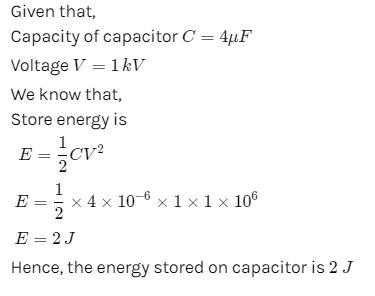QUESTION: 7

The energy of a charged capacitor resides in

Solution:
QUESTION: 8

The capacitance of a parallel plate condenser does not depend upon

Solution:
QUESTION: 9

The energy density in a parallel plate capacitor is given as 2.2 × 10-10 J/m3. The value of the electric field in the region between the plates is -

Solution:
QUESTION: 10

Two capacitances of capacity C1 and C2 are connected in series and potential difference V is applied across it. Then the potential difference acros C1 will be

Solution:
QUESTION: 11

A conductor of capacitance 0.5mF has been charged to 100volts. It is now connected to uncharged conductor of capacitance 0.2mF. The loss in potential energy is nearly

Solution:
QUESTION: 12

Two spherical conductors of capacitance 3.0mF and 5.0mF are charged to potentials of 300volt and 500volt. The two are connected resulting in redistribution of charges. Then the final potential is

Solution:
QUESTION: 13

A parallel plate capacitor of capacitance C is connected to a battery and is charged to a potential difference V. Another capacitor of capacitance 2C is similarly charged to a potential difference 2V. The charging battery is now disconnected and the capacitors are connect in parallel to each other in such a way that the positive terminal of one is connected to the negative terminal of the other. The final energy of the configuration is

Solution:
QUESTION: 14

A 2μF capacitor is charged to a potential = 10 V. Another 4μF capacitor is charged to a potential = 20V. The two capacitors are then connected in a single loop, with the positive plate of one connected with negative plate of the other. What heat is evolved in the circuit?

Solution:
*Multiple options can be correct
QUESTION: 15

The figure shows a diagonal symmetric arrangement of capacitors and a battery
Identify the correct statements.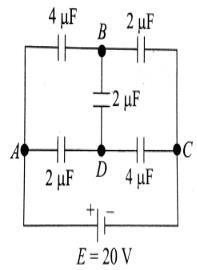Solution:
QUESTION: 16

In the circuit shown in figure charge stored in the capacitor of capacity 5μf is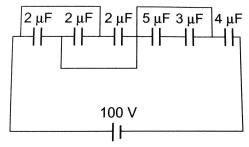Solution:
QUESTION: 17

Plate A of a parallel air filled capacitor is connected to a spring having force constant k and plate B is fixed. If a charge +q is placed on plate A and charge _q on plate B then find out extension in spring in equilibrim. Assume area of plate is `A'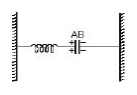Solution:
QUESTION: 18

Three uncharged capacitors of capacitane C1 = 1mF, C2 = 2mF and C3 = 3mF are connected as shown in figure to one another and to points A, B and D potential fA = 10V, fB = 25V and fD = 20 V, Determine the potential (f0) at point O.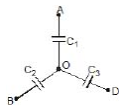Solution:
QUESTION: 19

Five capacitors are connected as shown in the figure. Initially S is opened and all capacitors are uncharged. When S is closed, steady state is obtained. Then find out potential difference between the points M and N.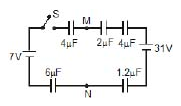Solution:
QUESTION: 20

Find the potential difference Va - Vb between the points a and b shows in each parts of the figure.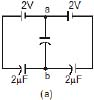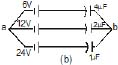Solution:
QUESTION: 21

Each plate of a parallel plate air capacitor has an area S. What amount of work has to be performed to slowly increase the distance between the plates from x1 to x2 If the charge of the capacitor, which is equal to q or

Solution:
QUESTION: 22

Each plate of a parallel plate air capacitor has an area S. What amount of work has to be performed to slowly increase the distance between the plates from x1 to x2 If the voltage across the capacitor, which is equal to V, is kept constant in the process.

Solution:
QUESTION: 23

If charge on left plane of the 5F capacitor in the circuit segment shown in the figure is-20C, the charge on the right plate of 3F capacitor is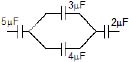Solution:
QUESTION: 24

In the circuit shown, the energy stored in 1μF capacitor is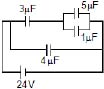Solution:
QUESTION: 25

A capacitor C1 = 4mF is connected in series with another capacitor C2 = 1mF. The combination is connected across a d.c. source of voltage 200V. The ratio of potential across C1 and C2 is

Solution:
QUESTION: 26

A 5.0 mF capacitor having a charge of 20 mC is discharged through a wire of resistance of 5.0 W. Find the heat dissipated in the wire between 25 to 50 ms after the capactions are made.

Solution:
QUESTION: 27

A charged capacitor is allowed to discharge through a resistance 2Ω by closing the switch S at the instant t = 0. At time t = ln2μs, the reading of the ammeter falls half of its initial value. The resistance of the ammeter equal to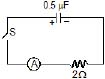Solution:
QUESTION: 28

A capacitor C =100μF is connected to three resistor each of resistance 1 kW and a battery of emf 9V. The switch S has been closed for long time so as to charge the capacitor. When switch S is opened, the capacitor. Discharges with time constant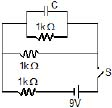Solution:
QUESTION: 29

In the transient shown the time constant of the circuit is :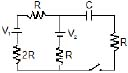Solution:
QUESTION: 30

In the circuit shown in figure C1 =2C2 . Switch S is closed at time t=0. Let i1 and i2 be the currents flowing through C1 and C2 at any time t, then the ratio i1 / i2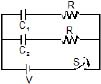Solution:
*Multiple options can be correct
QUESTION: 31

The two plates X and Y of a parallel plate capacitor of capacitance C are given a charge of amount Q each. X is now joined to the positive terminal and Y to the negative terminal of a cell of emf E = Q/C.

Solution:
*Multiple options can be correct
QUESTION: 32

Two capacitors of capacitances 1mF and 3mF are charged to the same voltages 5V. They are connected in parallel with oppositely charged plates connected together. Then

Solution:
*Multiple options can be correct
QUESTION: 33

Each plate of a parallel plate capacitor has a charge q on it. The capacitor is now connected to a battery. Now,

Solution:
*Multiple options can be correct
QUESTION: 34

When a parallel plates capacitor is connected to a source of constant potential difference,

Solution:
*Multiple options can be correct
QUESTION: 35

When two identical capacitors are charged individually to different potentials and connected parallel to each other, after disconnecting them from the source

Solution:
*Multiple options can be correct
QUESTION: 36

Two thin conducting shells of radii R and 3R are shown in the figure. The outer shell carries a charge +Q and the inner shell is neutral. The inner shell is earthed with the help of a switch S.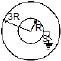Solution:
*Multiple options can be correct
QUESTION: 37

Two capacitors of 2mF and 3mF are charged to 150 volt and 120 volt respectively. The plates of capacitor are connected as shown in the figure. A discharged capacitor of capacity 1.5 mF falls to the free ends of the wire. Then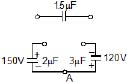Solution:
*Multiple options can be correct
QUESTION: 38

In the circuit shown initially C1, C2 are uncharged. After closing the switch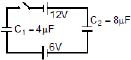Solution:
*Multiple options can be correct
QUESTION: 39

A circuit shown in the figure consists of a battery of emf 10V and two capacitance C1 and C2 of capacitances 1.0mF and 2.0mF respectively. The potential difference VA _ VB is 5V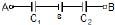Solution:
*Multiple options can be correct
QUESTION: 40

Two capacitors of equal capacitance (C1 = C2) are shown in the figure. Initially, while the switch S is open, one of the capacitors is uncharged and the other carries charge Q0. The energy stored in the charged capacitor is U0. Sometimes after the switch is colsed, the capacitors C1 and C2 carry charges Q1 and Q2, respectively, the voltages across the capacitors are V1 and V2, and the energies stored in the capacitors are U1 and U2. Which of the following statements is INCORRECT ?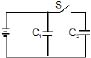Solution:
*Multiple options can be correct
QUESTION: 41

The figure shows a diagonal symmetric arrangement of capacitors and a battery

Identify the correct statements.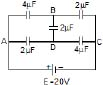Solution:
*Multiple options can be correct
QUESTION: 42

The figure shows a diagonal symmetric arrangement of capacitors and a batteryIf the potential of C is zero, then

Solution:
QUESTION: 43

For very low frequencies, capacitor acts as _______

Solution:

Capacitive impedance is inversely proportional to frequency. Hence at very low frequencies the impedance is almost infinity and hence acts as an open circuit and no current flows through it.

*Multiple options can be correct
QUESTION: 44

The charge across the capacitor in two different RC circuits 1 and 2 are plotted as shown in figure.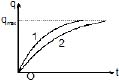Choose the correct statement (s) related to the two circuits

Solution:
*Multiple options can be correct
QUESTION: 45

The charge across the capacitor in two different RC circuits 1 and 2 are plotted as shown in figure.Identify the correct statement(s) related to the R1, R2, C1 and C2 of the two RC circuits.

Solution: